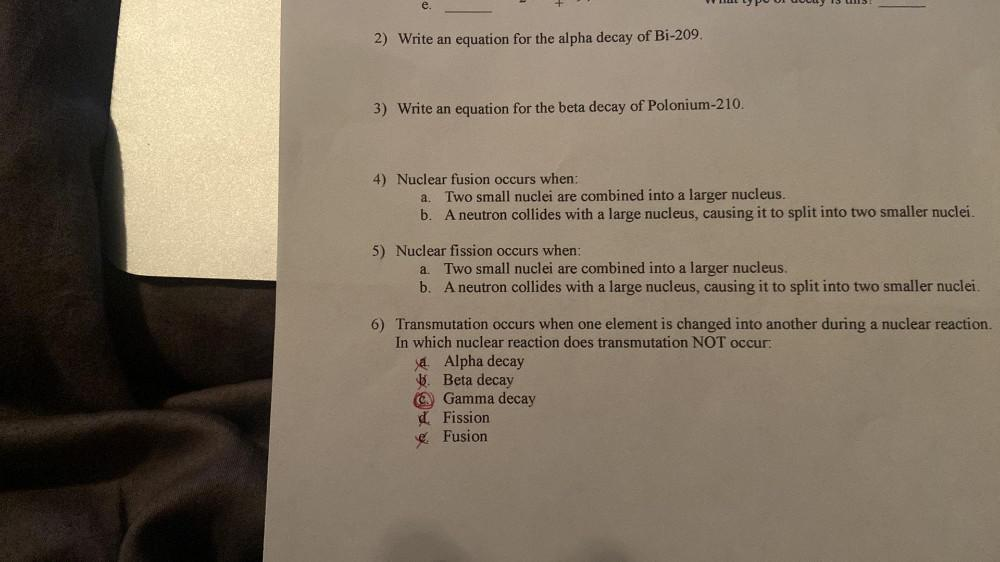Question:

# e. 2) Write an equation for the alpha decay of Bi-209. 3) Write an equation for the beta decay of Polonium-210. a. 4) Nuclear fue. 2) Write an equation for the alpha decay of Bi-209. 3) Write an equation for the beta decay of Polonium-210. a. 4) Nuclear fusion occurs when: Two small nuclei are combined into a larger nucleus. b. A neutron collides with a large nucleus, causing it to split into two smaller nuclei. a. 5) Nuclear fission occurs when: Two small nuclei are combined into a larger nucleus. b. A neutron collides with a large nucleus, causing it to split into two smaller nuclei. 6) Transmutation occurs when one element is changed into another during a nuclear reaction. In which nuclear reaction does transmutation NOT occur: Ja. Alpha decay b. Beta decay Gamma decay dFission Fusion C